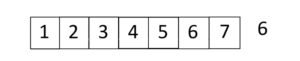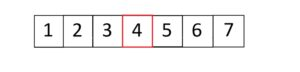# How to make a binary search in c#### Binary search in C#

Binary search is a powerful algorithm that allows you to quickly search for a specific value in a sorted array or list. This article will discuss how to implement a binary search in C# using the Array.BinarySearch() method, the IComparable interface, and the custom implementation of the binary search algorithm.

### Built-in Array.BinarySearch() method

To begin, let’s take a look at the Array.BinarySearch() method. This method is a built-in C# method that can be used to perform a binary search on an array. It takes two parameters: the array you want to search and the value you are looking for. It returns the index of the value if it is found, or a negative number if it is not found.

FLASH SALES
\$29.95
NEW
\$249.00

Here is an example of how to use the Array.BinarySearch() method:

This example creates an array of integers and searches for the number 5. The output of this program will be “The number 5 is at index 4”, which is the index of the number 5 in the array.

### Visual representation of the binary search algorithm

Binary search can be visualized as a process of repeatedly dividing a search interval in half.

1. The algorithm starts by comparing the middle element of the array or list with the target value.
2. If the target value is equal to the middle element, the search is complete and the index of the element is returned.
3. If it is less than the middle element, the search continues in the lower half of the array or list.
4. Otherwise, if the target value is greater than the middle element, the search continues in the upper half of the array or list.

Imagine we have a sorted array, and we want to find the index of 6 in itThe first step will be to pick the middle value and check if it equals 6. If yes, we are done in one step.

Otherwise, we must check whether it is smaller or bigger than 6. If it is smaller, we will follow with the right side in the other case, we take a left.

In our example, 6 is bigger than 4. That means we will take the right part.With this part, we have to repeat our manipulations. As you see, the middle value is 6. That means we are done with our search.### A custom binary search in C#

Another way to perform a binary search in C# is to use the IComparable interface. The IComparable interface is a built-in C# interface that allows you to define how to compare two objects of a certain type. By implementing this interface, you can create a custom binary or any other search algorithm that can be used with any type of object.

Here is an example of how to use the IComparable interface:

Here is the actual algorithm:

In this example, a custom class named “Person” is created with two properties, “Name” and “Age”. It implements the IComparable interface and defines the CompareTo method. The CompareTo method compares the ages of two Person objects. A binary search algorithm is then implemented that uses the CompareTo method to compare Person objects. The code then creates an array of Person objects and searches for a Person with an age of 32.